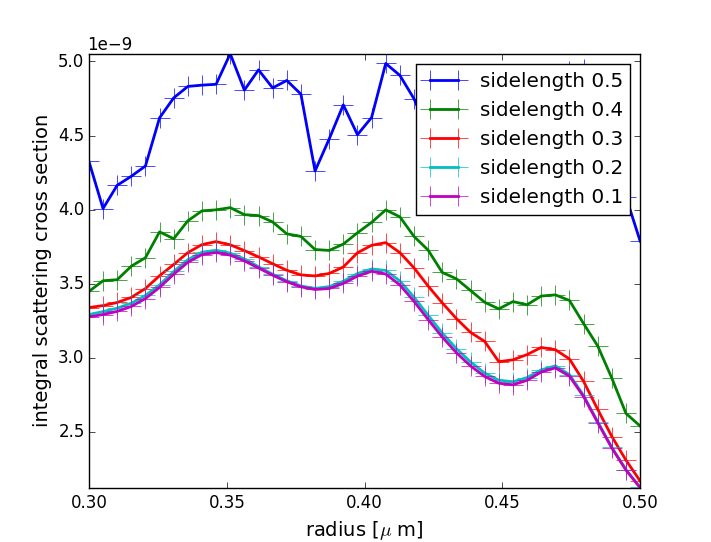# Persistent Parameter Scan¶

For complex setups one often wants to run different (sometimes multi-dimensional) parameter scans. In this case it is worthwhile to store the results of parameter scans such that they don’t have to be recalculated in future parameter scans. To manage computational results one can make use of the class jcmwave.Resultbag.

We revisit the example of a parallel parameter scan (Parallel Parameter Scan). As before we start by going into daemon mode:

jcmwave.daemon.shutdown()
Multiplicity = 2,


The project files in the current example do not only depend on the rod radius but also on the two additional parameters finite_element_degree (used in mie2D.jcmpt) and maximum_sidelength (used in layout.jcmt). In general, not all parameters are varied during a parameter scan. Therefore, we define a set of default parameters:

default_keys = {
'finite_element_degree': 3,
'maximum_sidelength': 0.1,
}


Next, we initialize a resultbag by calling jcmwave.Resultbag with a filepath as parameter. The data of the resultbag will be stored to this file. If it already exists all data from this file is loaded into the resultbag.

resultbag = jcmwave.Resultbag('resultbag.db')


Warning

If you use more than one resultbag make sure that each resultbag stores its results to a different file.

With the help of NumPy’s meshgrid-function we can define a variable keyset that holds a two-dimensional parameter scan over the parameters maximum_sidelength and radius:

import numpy.matlib as matlib
from copy import copy

sidelengths = np.linspace(0.5, 0.1, 5)
keyset = matlib.tile({},*scan1.shape)
for ii in np.ndindex(*scan1.shape):
keyset[ii] = copy(default_keys)
keyset[ii]['maximum_sidelength'] = scan1[ii]


The structure can be easily extended to n-dimensional parameter scans by introducing additional scan grids scan3, scan4, etc.

Next, we run in parallel all the computations that are defined by the keyset:

job_ids = []

for ii in np.ndindex(*keyset.shape):
keys = keyset[ii]
job_id = jcmwave.solve('mie2D.jcmp', keys = keys,
temporary = True,
resultbag = resultbag)
job_ids.append(job_id)

jcmwave.daemon.wait(job_ids, resultbag = resultbag)


By calling the solver command with the resultbag as the third parameter jcmwave.solve() checks if the computation for the project files (mie2D.jcmpt, layout.jcmt, materials.jcm, sources.jcm) and the current keys is already contained in the resultbag. If this is the case, the computation is automatically skipped. Otherwise the new result is added to the resultbag by the function jcmwave.daemon.wait() to which the resultbag is passed as the second parameter. Whenever a result is added it is also stored to the disk.

Note

Sometimes a computation does not depend on all parameters in the keys-structure (e. g. a waveguide-mode computation generally depends on fewer parameters than a subsequent scattering computation that uses a waveguide mode as light source). We can account for this by calling jcmwave.Resultbag with a second parameter that defines the fieldname of all relevant parameters. For example by initializing the resultbag as

resultbag = jcmwave.Resultbag('resultbag.db',['finite_element_degree', 'radius'])


a change of the parameter maximum_sidelength would not trigger a new computation.

For given keys the results and corresponding logs of a computation can be accessed by calling result = resultbag.get_result(keys) and log = resultbag.get_log(keys) respectively. A plot of the scattering cross section against the rod radius for different maximum sidelengths can be produced in the following way (see Figure “Scattering Cross Section”):

scattering_cross_section_scan = np.zeros(keyset.shape)
for ii in np.ndindex(*keyset.shape):
keys = keyset[ii]
result = resultbag.get_result(keys)
scs = result['ElectromagneticFieldEnergyFlux'].real
scattering_cross_section_scan[ii] = scs

import matplotlib.pyplot as plt
handles = []
for data in zip(*scattering_cross_section_scan):
handle, = plt.plot(radii, data, '-+', linewidth=2, markersize=14)
handles.append(handle)
plt.legend(handles, ['sidelength %.1f' % s for s in sidelengths])
plt.xlabel('radius [$\mu$ m]', fontsize=14)
plt.ylabel('integral scattering cross section', fontsize=14)
plt.axis('tight')
plt.show()Scattering Cross Section

Computed scattering cross section versus rod radius for different maximum sidelengths.